# NumPy: reshape() function

## numpy.reshape() function

The reshape() function is used to give a new shape to an array without changing its data.

Syntax:

`numpy.reshape(a, newshape, order='C')`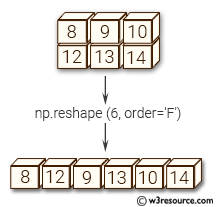Version: 1.15.0

Parameter:

Name Description Required /
Optional
a Array to be reshaped. Required
newshape The new shape should be compatible with the original shape. If an integer, then the result will be a 1-D array of that length. One shape dimension can be -1. In this case, the value is inferred from the length of the array and remaining dimensions. Required
order Read the elements of a using this index order, and place the elements into the reshaped array using this index order. ‘C’ means to read / write the elements using C-like index order, with the last axis index changing fastest, back to the first axis index changing slowest. ‘F’ means to read / write the elements using Fortran-like index order, with the first index changing fastest, and the last index changing slowest. Note that the ‘C’ and ‘F’ options take no account of the memory layout of the underlying array, and only refer to the order of indexing. ‘A’ means to read / write the elements in Fortran-like index order if a is Fortran contiguous in memory, C-like order otherwise. Optional

Return value:

reshaped_array : ndarray - This will be a new view object if possible; otherwise, it will be a copy. Note there is no guarantee of the memory layout (C- or Fortran- contiguous) of the returned array.

Example-1: numpy.reshape() function

``````>>> import numpy as np
>>> x = np.array([[2,3,4], [5,6,7]])
>> np.reshape(x, (3, 2))
array([[2, 3],
[4, 5],
[6, 7]])
``````

Pictorial Presentation: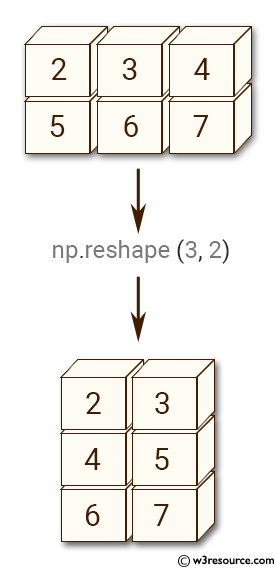Example-2: numpy.reshape() function

``````>>> import numpy as np
>>> x = np.array([[2,3,4], [5,6,7]])
>>> np.reshape(x, (2, -2))
array([[2, 3, 4],
[5, 6, 7]])

``````

Pictorial Presentation: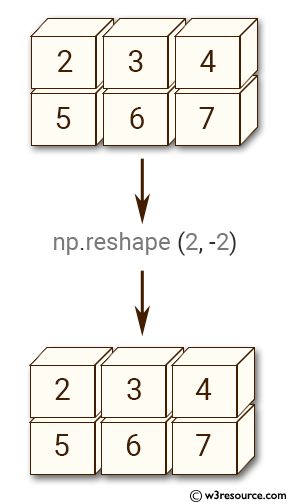Example-3: numpy.reshape() function

``````>>> import numpy as np
>>> x = np.array([[2,3,4], [5,6,7]])
>>> np.reshape(x, (3, -1))
array([[2, 3],
[4, 5],
[6, 7]])
``````

Pictorial Presentation: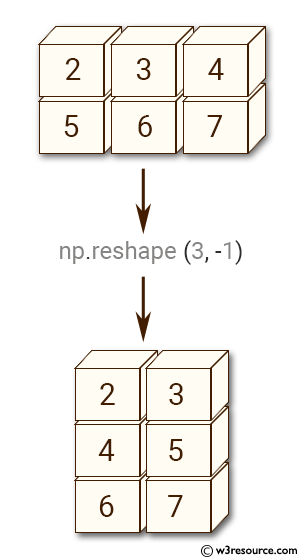Example-4: numpy.reshape() function

``````>>> import numpy as np
>>> x = np.array([[2,3,4], [5,6,7]])
>>> np.reshape(x, 6)
array([2, 3, 4, 5, 6, 7])
``````

Pictorial Presentation: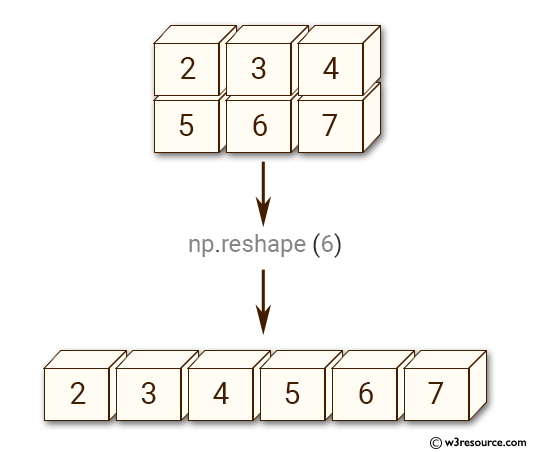Example-5: numpy.reshape() function

``````>>> import numpy as np
>>> x = np.array([[2,3,4], [5,6,7]])
>>> np.reshape(x, 6, order='F')
array([2, 5, 3, 6, 4, 7])
``````

Python - NumPy Code Editor:

Previous: copyto()
Next: ravel()

﻿